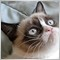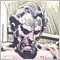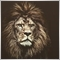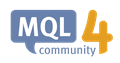# How to give an indicator my own data?963

For example if I want to give the EMA a custom value instead of iCLose or the default values so I can see into the future, how can I do this? Do I have to reverse engineer MetaQuotes source or code a brand new complicated array indicator just for that?  How would you go about it for  a simple task? I just want to calculate what price is Bid going to be when MACD is at 0 line however I need to calculate EMAs to do this. I just need a simpe Price to EMA script, however I only have

```//+------------------------------------------------------------------+
//|                                                      ProjectName |
//|                                       http://www.companyname.net |
//+------------------------------------------------------------------+
#property indicator_chart_window
#property indicator_buffers 1

double MA_Period12=12;
int MA_Shift12 = 0;
int SetPrice12 = 0;
double ExtMapBuffer12[];
double ema12;
int ExtCountedBars12=0;
//+------------------------------------------------------------------+
int init()
{
IndicatorDigits(MarketInfo(Symbol(),MODE_DIGITS));
SetIndexShift(0,MA_Shift12);
SetIndexBuffer(0,ExtMapBuffer12);
//SetIndexStyle(0,DRAW_NONE);
}
//+------------------------------------------------------------------+
int start()
{

if(Bars <= MA_Period12) return(0);
ExtCountedBars12=IndicatorCounted();
if(ExtCountedBars12 < 0) return(-1);
if(ExtCountedBars12>0) ExtCountedBars12--;
//----
ema12_function();
double pr12;
pr12=2.0/(MA_Period12+1);
int pos12=Bars-2;
if(ExtCountedBars12>2) pos12=Bars-ExtCountedBars12-1;
//---- main calculation loop
while(pos12>=0)
{
if(pos12==Bars-2)
ExtMapBuffer12[pos12+1]=(Open[pos12+1]+Close[pos12+1])/2;
ExtMapBuffer12[pos12]=Bid*pr12+ExtMapBuffer12[pos12+1]*(1-pr12);
pos12--;
ema12=ExtMapBuffer12[pos12];
Print ("GetPrice12(pos12) = ",GetPrice12(pos12));
Print("Bid = " , Bid);
Print("ema12 = ",ema12);
Print("default ema 12 = ",iMA(_Symbol,PERIOD_M1,12,0,MODE_EMA,PRICE_CLOSE,0));
//   Print("default ema 26 = ", iMA(_Symbol,PERIOD_M1,26,0,MODE_EMA,PRICE_CLOSE,0));
return(0);
}
}
//----
void ema12_function()
{
double pr12;
pr12=2.0/(MA_Period12+1);
int pos12=Bars-2;
if(ExtCountedBars12>2) pos12=Bars-ExtCountedBars12-1;
//---- main calculation loop
while(pos12>=0)
{
if(pos12==Bars-2)
ExtMapBuffer12[pos12+1]=(Open[pos12+1]+Close[pos12+1])/2;
ExtMapBuffer12[pos12]=GetPrice12(pos12)*pr12+ExtMapBuffer12[pos12+1]*(1-pr12);
pos12--;
}
}
//+------------------------------------------------------------------+
double GetPrice12(int shift12)
{
double price12;
price12=Close[shift12];
return(price12);
}
//+------------------------------------------------------------------+
```

A lot harder than I expected, I have to now assemble a perfect replica of MetaQuotes source code that takes my input data, is this even going to work or is it going to be clunky at the end for some reason? Ive had weird things with this MQL where they just tell me to give it up, is this one of them? Can be done I assume, however it is acting weird because it seems to rely on array version of Bid data, stored in EXTMAPBUFFER as position values. Not an expert as you can tell. If you just put Bid there it works then it starts acting up. Whats the next step here? Any ideas?

As a side note, do you know how to build the exact YSCALE formula? I tried measuring my screen with on screen ruler and cannot get their formula down. I can get size of pixel, and pixel count between one point of monitor with the other with an awesome linux accesory, and I cannot get any answer that is not NO CAN DO ?3259

Well, EMA is just a formula that takes three inputs and spits out an output.

This macro can simplify your code, but in essence, it's the same formula you have above.

```// P == Previous EMA value, i.e. EMA of previous day's price
// C == Current value (e.g. close price)
// L == Time period, e.g. a 10 day moving average
// Example: MaBuffer0[i]=EMA(MaBuffer0[i-1], dCurrValue, g_maPeriod);
#define EMA(P, C, L) ((P) + (2.0/((L)+1.0))*((C)-(P)))```

I use this formula all the time.

There is a library of MQL5 routines, one of which should also fit your needs.

https://www.mql5.com/en/code/77

But yes, if you want to create an indicator that does EMA on your terms, I would probably start with the free indicator from MetaQuotes and tweak it until it fits your needs.9826

For example if I want to give the EMA a custom value instead of iCLose or the default values so I can see into the future, how can I do this? Do I have to reverse engineer MetaQuotes source or code a brand new complicated array indicator just for that?  How would you go about it for  a simple task? I just want to calculate what price is Bid going to be when MACD is at 0 line however I need to calculate EMAs to do this. I just need a simpe Price to EMA script, however I only have

A lot harder than I expected, I have to now assemble a perfect replica of MetaQuotes source code that takes my input data, is this even going to work or is it going to be clunky at the end for some reason? Ive had weird things with this MQL where they just tell me to give it up, is this one of them? Can be done I assume, however it is acting weird because it seems to rely on array version of Bid data, stored in EXTMAPBUFFER as position values. Not an expert as you can tell. If you just put Bid there it works then it starts acting up. Whats the next step here? Any ideas?

As a side note, do you know how to build the exact YSCALE formula? I tried measuring my screen with on screen ruler and cannot get their formula down. I can get size of pixel, and pixel count between one point of monitor with the other with an awesome linux accesory, and I cannot get any answer that is not NO CAN DO ?

if you want to give custom values for EMA (for any MA for that matter) use iMAOnArray()

https://docs.mql4.com/indicators/imaonarrayiMAOnArray - Technical Indicators - MQL4 Reference
• docs.mql4.com
Unlike iMA(...), the iMAOnArray() function does not take data by symbol name, timeframe, the applied price. The price data must be previously prepared. The indicator is calculated from left to right. To access to the array elements as to a series array (i.e., from right to left), one has to use the ArraySetAsSeries() function.963

Lakshan Perera:

if you want to give custom values for EMA (for any MA for that matter) use iMAOnArray()

https://docs.mql4.com/indicators/imaonarray

How do I give it for example, Bid + 1*Digit as input value for EMA to iOnArray? That even possible?963

How do I give it for example, Bid + 1*Digit as input value for EMA to iOnArray? That even possible?

pass custom value to ima on array?

```double  iMAOnArray(
double       array[],          // array with data
int          total,            // number of elements
int          ma_period,        // MA averaging period
int          ma_shift,         // MA shift
int          ma_method,        // MA averaging method
int          shift             // shift
);```

looks like it can take an array that I create. So I just make an array with the Current price. It will not work. i need to create an array that includes the new bid +1 array? Makes little sense to use this.963

double  iMAOnArray(
double       array[],          // array with data
int          total,            // number of elements
int          ma_period,        // MA averaging period
int          ma_shift,         // MA shift
int          ma_method,        // MA averaging method
int          shift             // shift
);

Instead of Ma Shift, How do I change to Custom value like bid+1 ?

I apparently need an array for current bid price, with a bunch of closes for previous candles up to candle 12 because I need a 12 ema.9826

How do I give it for example, Bid + 1*Digit as input value for EMA to iOnArray? That even possible?

I did not understand well what did you meant by Bid + 1*Digit ,
but in order to pass custom values to the iMAOnArray() , first you have to prepare an array which include the custom price data that you want to input to the function..963

Lakshan Perera:

I did not understand well what did you meant by Bid + 1*Digit ,
but in order to pass custom values to the iMAOnArray() , first you have to prepare an array which include the custom price data that you want to input to the function..

double ExponentialMA(const int position,const int period,const double prev_value,const double &price[])
{
//---
double result=0.0;
//--- calculate value
if(period>0)
{
double pr=2.0/(period+1.0);
result=price[position]*pr+prev_value*(1-pr);
}
//---
return(result);
}

Here do I put the bid price here to get a custom EMA of whatever input Bid? Like bid +20*Digit and Custom random input values to calculate the EMA etc?963

Anthony Garot:

Well, EMA is just a formula that takes three inputs and spits out an output.

This macro can simplify your code, but in essence, it's the same formula you have above.

I use this formula all the time.

There is a library of MQL5 routines, one of which should also fit your needs.

https://www.mql5.com/en/code/77

But yes, if you want to create an indicator that does EMA on your terms, I would probably start with the free indicator from MetaQuotes and tweak it until it fits your needs.

Does this give the exact perfect 7 digiits?963

`prev_value I just put whatever I want in that variable?`
I have to see into future by adding custom values to ema so i can now what its going to be in the future.963

Lakshan Perera:

I did not understand well what did you meant by Bid + 1*Digit ,
but in order to pass custom values to the iMAOnArray() , first you have to prepare an array which include the custom price data that you want to input to the function..

So I generate array and supply all  of the closes? Should go with source built MapExt Stuff?

How wull I put custom data in it?

Incredible when all I need is somewhere to be able to put my custom bid +1 possibilities. Should be able to put different numbers somewhere without incorrect at the fifth decimal.

```double  iMA(
string       symbol,           // symbol
int          timeframe,        // timeframe
int          ma_period,        // MA averaging period
int          ma_shift,         // MA shift
int          ma_method,        // averaging method
int          applied_price,    // applied price
int          shift             // shift
);```

however this is a lot more complicated because I need 2 arrays however I cant have an array of the custom price. Its just assuming if price was bid +1 or bid +2 etc so as to predict where the MACD crosses 0 so I can put a pending order on the price instead of normal laggy market orders.